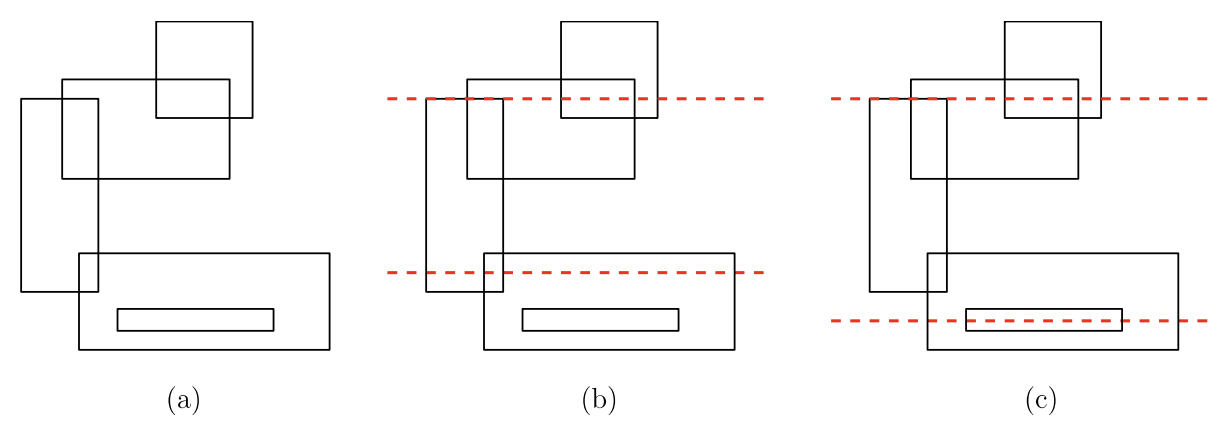시간 제한메모리 제한제출정답맞힌 사람정답 비율
2 초 512 MB102626021027.851%

## 문제

There are a number of electronic circuits, such as CPU’s, ROM’s, RAM’s, to be printed in a single chip consisting of multiple layers. Due to some design restriction, there can be only two electrical wires that are horizontal segments. Your job is to find two horizontal wires that together connect as many circuits as possible so that the electric signals go through the circuits.

This problem can be stated formally as follows. There are n axis-aligned rectangles in the plane. Each of the rectangles represents a circuit to be printed in the chip. The rectangles may overlap each other. You are supposed to find two horizontal lines such that the total number of rectangles intersected by the two lines is maximized. We also say that a rectangle is intersected by a horizontal line if the line contains the top side or the bottom side of the rectangle. If a rectangle is intersected by both the lines, it is counted only once for the total number.

For example, let’s consider 5 rectangles shown in Figure A.1. Figure A.1(c) shows two horizontal lines (red dashed lines) that intersect all 5 rectangles while the two horizontal lines (red dashed lines) in Figure A.1(b) intersect 4 rectangles.Figure A.1: (a) 5 axis-aligned rectangles. (b) Two horizontal lines that intersect 4 rectangles. (c) Two horizontal lines that intersect 5 rectangles.

Given a set of axis-aligned rectangles, write a program to find two horizontal lines such that the total number of rectangles intersected by the two lines is maximized.

## 입력

Your program is to read from standard input. The first line contains a positive integer n representing the number of axis-aligned rectangles in the plane, where 3 ≤ n ≤ 100,000. It is followed by n lines, each contains four integers ux, uy, vx and vy (with ux < vx and uy > vy) representing the (x, y)-coordinates, (ux, uy), of the top-left corner and the (x, y)-coordinates, (vx, vy), of the bottom-right corner of an axisaligned rectangle, where −10,000,000 ≤ ux, uy, vx, vy ≤ 10,000,000.

## 출력

Your program is to write to standard output. Print exactly one line. The line should contain the maximum total number of rectangles that can be intersected by two horizontal lines.

## 예제 입력 1

5
0 13 4 4
2 14 11 9
7 17 12 12
3 5 16 0
5 2 13 1


## 예제 출력 1

5


## 예제 입력 2

5
0 4 4 0
1 3 3 1
5 8 9 4
0 12 4 8
1 11 3 9


## 예제 출력 2

4